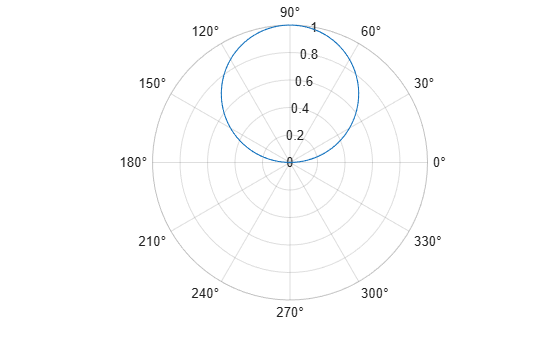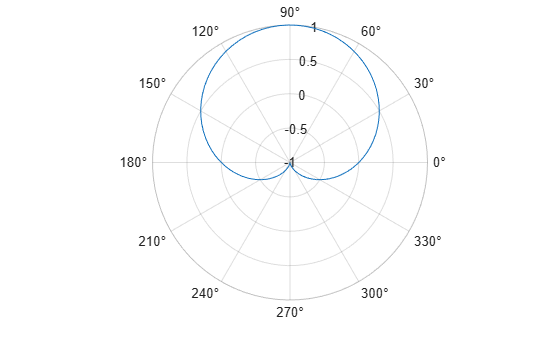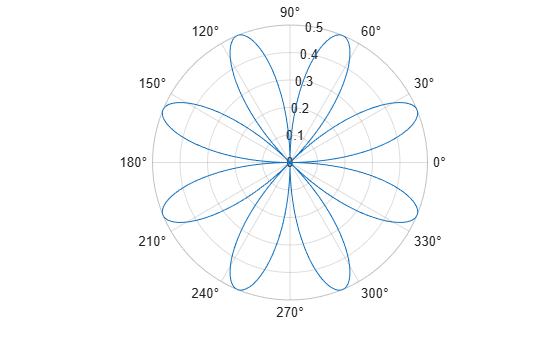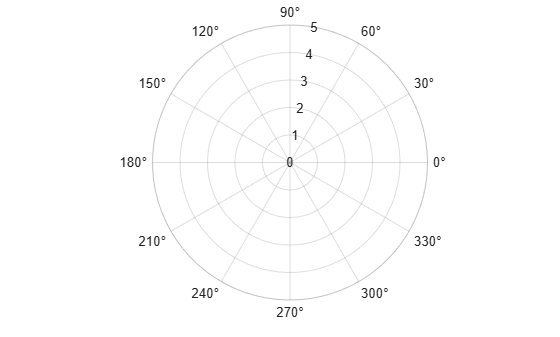rlim

Set or query r-axis limits for polar axes

Description

example

rlim(limits) specifies the r-axis limits for the current polar axes. Specify limits as a two-element vector of the form [rmin rmax], where rmax is a numeric value greater than rmin.

example

rlim('auto') lets MATLAB® choose the r-axis limits. This command sets the RLimMode property for the polar axes object to 'auto'.

rlim('manual') prevents the limits from changing automatically. Use this option if you want to retain the current limits when adding new data to the polar axes using the hold on command. This command sets the RLimMode property for the polar axes object to 'manual'.

example

rl = rlim returns a two-element vector containing the limits for the current polar axes.

m = rlim('mode') returns the current value of the limits mode, which is either 'auto' or 'manual'. By default, the mode is automatic unless you specify limits or set the mode to manual.

example

___ = rlim(pax,___) uses the polar axes specified by pax instead of the current polar axes. Specify pax as the first input argument. Include additional input or output arguments only if the original syntax supported them.

Examples

collapse all

Create a polar plot and change the r-axis limits.

theta = 0:0.01:2*pi;
rho = sin(2*theta).*cos(2*theta);
polarplot(theta,rho)
rlim([0 1])Set the limits back to the original values.

rlim('auto')Create a polar plot using negative radius values. By default, polarplot reflects negative values through the origin.

theta = linspace(0,2*pi);
rho = sin(theta);
polarplot(theta,rho)Change the limits of the r-axis so it ranges from -1 to 1.

rlim([-1 1])Create a polar plot and return the r-axis limits.

theta = 0:0.01:2*pi;
rho = sin(2*theta).*cos(2*theta);
polarplot(theta,rho)rl = rlim
rl = 1×2

0    0.5000

Set the limits for a specific polar axes by specifying the polar axes object as the first input to rlim. Otherwise, rlim sets the limits for the current axes.

pax = polaraxes;
rlim(pax,[0 5])Input Arguments

collapse all

Minimum and maximum limits, specified as a two-element vector of the form [rmin rmax], where rmax is a numeric value greater than rmin. You can specify both limits, or specify one limit and let MATLAB automatically calculate the other.

• To automatically set the minimum limit to the minimum data value, specify the first element as -inf, for example, rlim([-inf 0]).

• To automatically set the maximum limit to the maximum data value, specify the second element as inf, for example, rlim([0 inf]).

When you specify the limits, the RLim property for the polar axes object updates to the specified values and the RLimMode property changes to 'manual'.

Example: rlim([0 1])

Polar axes, or an array of polar axes. If you do not specify this argument, then rlim sets the limits for the current axes (provided that the current axes is a polar axes object).

Output Arguments

collapse all

Current limits, returned as a two-element vector of the form [rmin rmax]. Querying the limits returns the value of the RLim property for the polar axes object.

Current limits mode, returned as one of these values:

• 'auto' — The limits automatically update to reflect changes in the data.

• 'manual' — The limits do not automatically update to reflect changes in the data.

Querying the r-axis limits mode returns the value of the RLimMode property for the polar axes object.